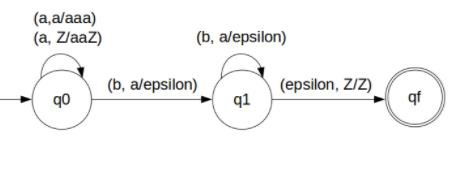# Construct a DPDA for anb2n n ‚â• 1 in TOC

A deterministic finite automata (DFA) can remember a finite amount of information but A push down automata (PDA) can remember an infinite amount of information.

Basically a PDA is as follows −

“Finite state machine+ a stack”

PDA has three components, which is as follows −

• An Input tape
• A control unit
• A Stack with infinite size

A PDA can be formally described as seven tuples (Q, Σ,S, δ,q0,I,F)

• Q is finite number of states
• Σ is input alphabet
• S is stack symbol
• Δ is the transition function: QX(Σ∪{e})XSXQ
• q0 is the initial state (q0 belongs to Q)
• I is the initial state top symbol
• F is a set of accepting states

## Problem

Construct PDA for anb2n n ≥ 1.

## Solution

So, the strings which are generated by the given language are as follows −

L={abb,aabbbb,aaabbbbbb,….}

Here a’s are followed by double the b’s

Whenever ‘a’ comes, push ‘a’ two times in the stack and if ‘a’ comes again then do the same.

When ‘b’ comes then pop one ‘a’ from the stack each time. Note that b comes after ‘a’.

Finally at the end of the strings, if nothing is left in the STACK, then we can declare that language is accepted in the PDA.

The PDA for the problem is as follows −## Transition functions

The transition functions are as follows −

Step 1: δ(q0, a, Z) = (q0, aaZ)

Step 2: δ(q0, a, a) = (q0, aaa)

Step 3: δ(q0, b, a) = (q1, ε)

Step 4: δ(q1, b, a) = (q1, ε)

Step 5: δ(q1, ε, Z) = (qf, Z)

## Explanation

Step 1 − Consider input string: "aabbbb" which satisfies the given condition.

Step 2 − Scan string from left to right.

Step 3 − For input 'a' and STACK alphabet Z, then

Step 4 − For input 'a' and STACK alphabet 'a', then

Push the two 'a's into STACK: (a,a/aaa) and state will be q0. Now the STACK has "aaaa".

Step 5 − For input 'b' and STACK alphabet 'a', then

Pop one 'a' from STACK: (b,a/ε) and state will be q1.

Step 6 − For input 'b' and STACK alphabet 'a' and state q1, then

Pop one 'a' from STACK: (b,a/ε) and state will remain q1

Step 7 − For input 'b' and STACK alphabet 'a', then

Pop one 'a' from STACK: (b,a/ε) and state will be q1

Step 8 − For input 'b' and STACK alphabet 'a' and state q1, then

Pop one 'a' from STACK: (b,a/ε) and state will remain q1

Step 9 − We reached end of the string, for input ε and STACK alphabet Z,

Go to final state(qf): (ε, Z/Z)